# Expressions and Equations

If you think about maths as a language, expressions and equations are the sentences. This unit brings students into the world of “maths language”, learning how to write complex expressions in different forms and convert numbers in one form to another (i.e.decimals to fractions). Last, students will apply the order of operations to interpret and solve simple algebraic equations.
This lesson includes 5 printable learning activities.

## Converting Numerical Expressions to Different FormsFor students who love word problems, show them how to convert numerical expressions to different forms.

## Order of Operations and use of Parentheses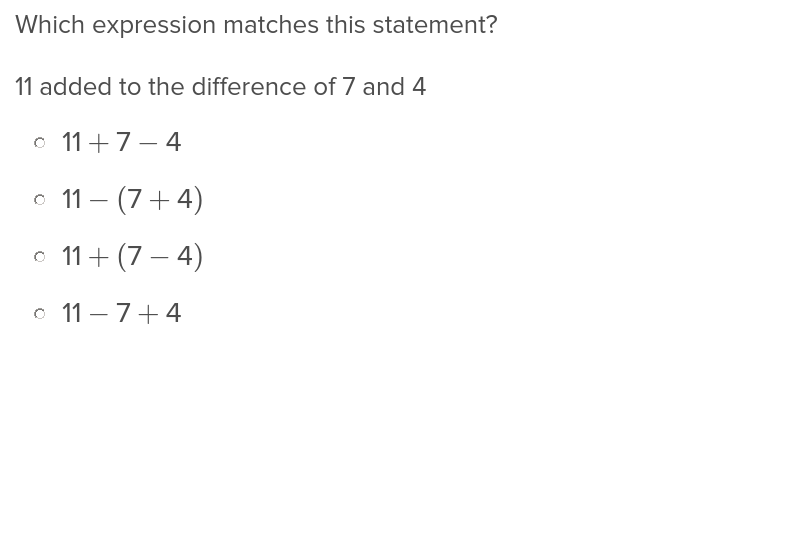Reinforce students’ understanding of PEMDAS by practising the order of operations and using parentheses in this exercise.

## Defeating the Mathemagician: Expressions, Equations, and Variables Story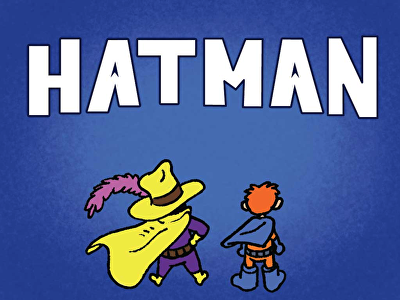Lucas, a superhero-in-training, learns all about expressions, equations, and variables to help Hatman on his quest in defeating the Mathemagician—but they can't do it alone! In this action-packed maths story, kids must answer multiple choice questions to move the story along. This interactive learning experience in which story meets game is an engaging way to boost your fifth grader’s understanding of expressions and equations.

## Understanding Expressions and Equations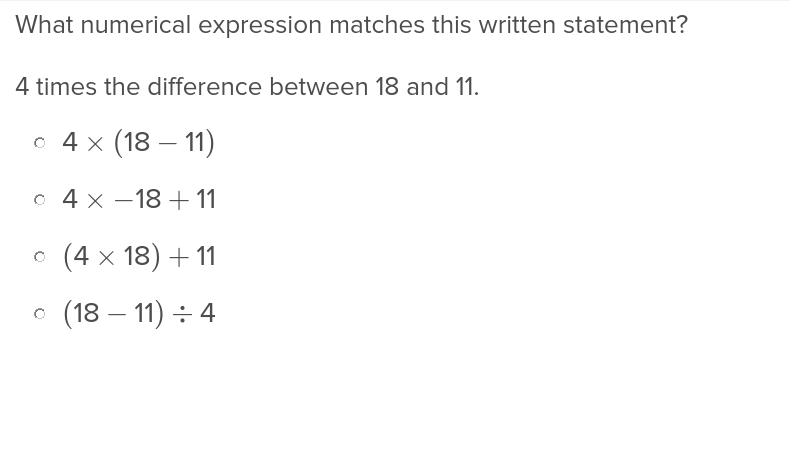Students will understand mathematical expressions and equations after they work through this engaging exercise from Education.com.

## Solving Basic Algebraic Equations: Basketball Edition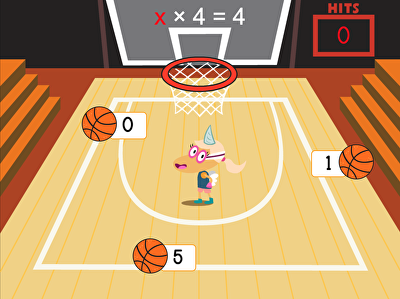Test your child's maths accuracy with action-packed game! Fifth graders can join Penelope as she dribbles, shoots, and scores her way across the court by solving basic algebraic equations. Working on a range of operations, from addition to division, this gets your child acquainted with algebra and starts them on the road to understanding expressions and equations.

## Solving Basic Algebraic Equations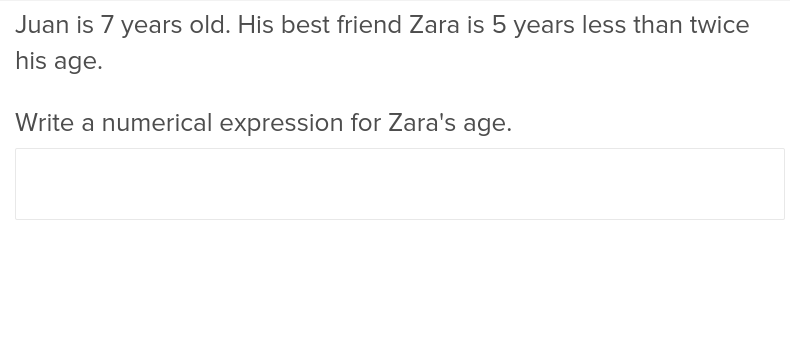Students will appreciate this exercise that shows how to solve basic algebraic equations with ease.

## Convert Decimals to Fractions 2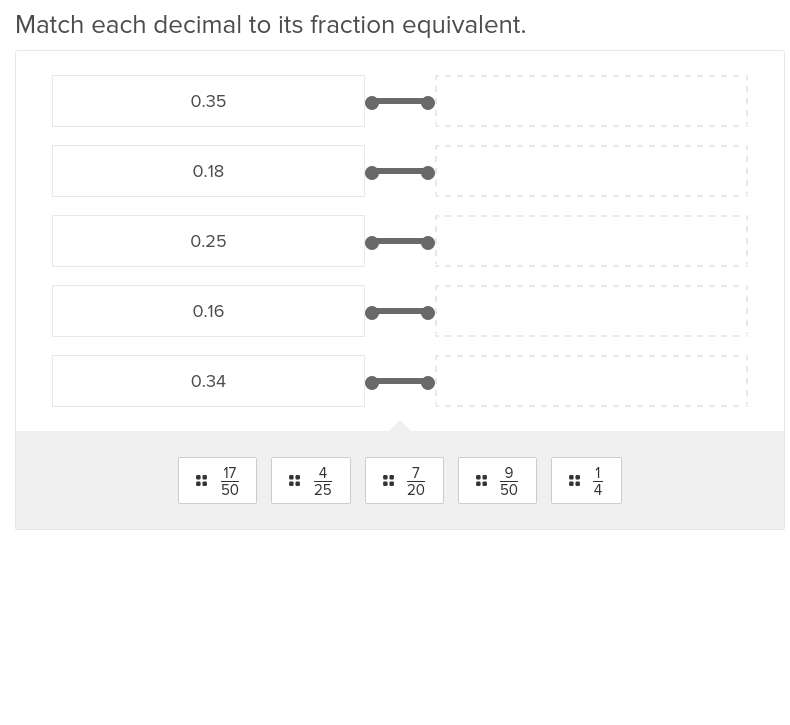Students will no longer be intimidated by converting decimals to fractions after this simple exercise.

Create new collection

0

### New Collection>

0Items

What could we do to improve Education.com?